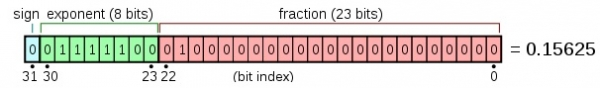# How to count set bits in a floating point number in C?

In this problem, one floating point value is given. We have to find number of set bits in the binary representation of it.

For example, if a floating point number is 0.15625, so there are six set bits. A typical C compiler used single precision floating point representation. So it will be look like this.To convert into its bit values, we have to take the number into one pointer variable, then typecast the pointer to char* type data. Then process each byte one by one. Then we can count set bits of each char.

## Example

#include <stdio.h>
int char_set_bit_count(char number) {
unsigned int count = 0;
while (number != 0) {
number &= (number-1);
count++;
}
return count;
}
int count_float_set_bit(float x) {
unsigned int n = sizeof(float)/sizeof(char); //count number of characters in the binary equivalent
int i;
char *ptr = (char *)&x; //cast the address of variable into char
int count = 0; // To store the result
for (i = 0; i < n; i++) {
count += char_set_bit_count(*ptr); //count bits for each bytes ptr++;
}
return count;
}
main() {
float x = 0.15625;
printf ("Binary representation of %f has %u set bits ", x, count_float_set_bit(x));
}

## Output

Binary representation of 0.156250 has 6 set bits